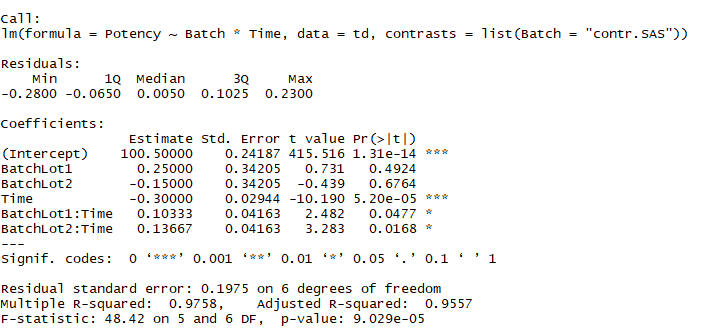Memu refer below

# 基于Minitab, JMP, SPSS, R做回归分析

• I：序贯型，嵌套设计
• II：平衡设计，仅主效应
• III：调整型
• IV：不完整数据

### Contrasts(对比):

• Minitab/JMP无此项
• R/SPSS有。

R中: options(contrasts=c(unordered="contr.treatment",ordered="contr.poly"))为默认选项。此选项在方差分析或回归分析建模时，如果有因子，可以使用此参数。第一个是无序因子的参数，第二个是有序因子的参数。同时，也可以通过在建模时指定contrasts参数。比如td_fit<-lm(Potency~Lot*Time,data=td,contrasts = list(Lot="contr.sum"))

• R（系数对比）:

- contr.helmert:
- contr.poly
- contr.SAS: SPSS默认使用此对比方式，设置此项时，R语言返回结果与SPSS的参数估计值相同。
- contr.sum: JMP默认使用此对比方式，设置此项时，R语言返回结果与JMP的协变量，交互效应，截距参数       估计值相同。
- contr.treatment：Minitab默认使用此对比方式，设置此项时，R语言返回结果与Minitab的参数估计值相       同。

• R(方差分析表）

- contr.helmert/contr.sum/contr.poly:设置此项时，R语言返回结果与SPSS的参数估计值相同。
- contr.SAS: 无具体描述
- contr.treatment：无具体描述


### 结果输出对比 (R vs JMP)

JMP 文件

CSV 数据文件

JMP的分析结果如下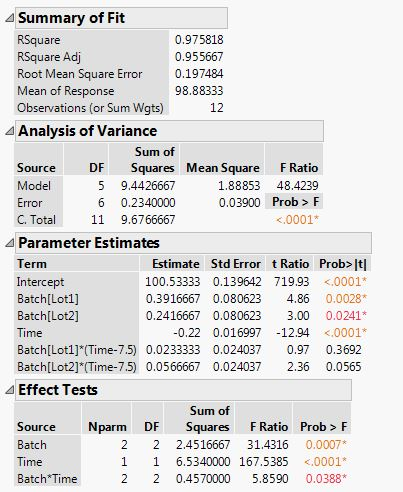R Code

td<-read.csv(”~/regression_interactive.csv”) td$time<-scale(td$Time,scale = FALSE,center = TRUE) td_fit<-lm(Potency~time,data=td,contrasts=list(Batch=“contr.sum”)) summary(td_fit) library(car) Anova(td_fit,type=“III”)

R的分析结果如下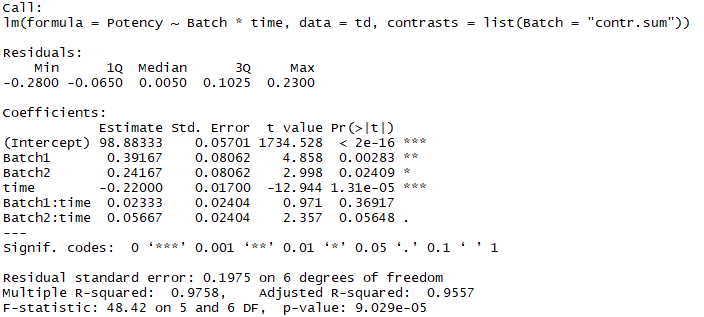#### 其中，比较重要的是对于无序因子的SS平方和计算的设置，要设置成"contr.sum",另外，应该把Time中心化，然后再拟合模型。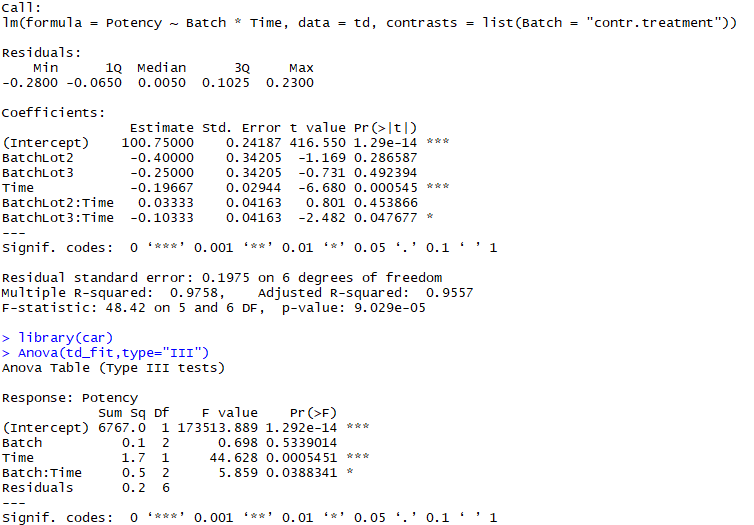slope=tan(A)=a/b, 其中a是截距变化，b是横坐标变化。

JMP虽然在计算系数的时候中心化了，但是计算截距的时候，还原成了真实截距。

### 结果输出对比 (R vs Minitab)

Minitab的分析结果如下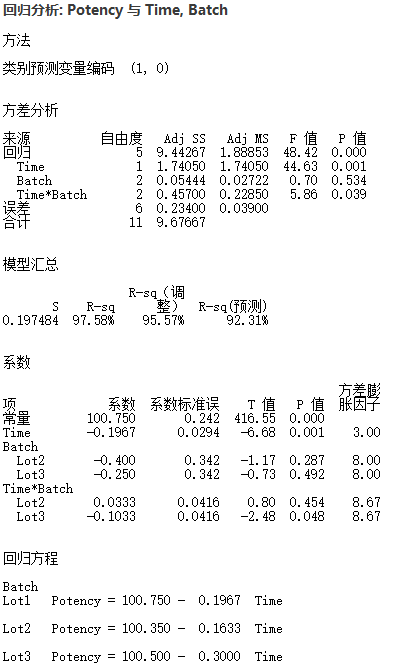R Code

R的分析结果如下### 结果输出对比 (R vs SPSS)

#### 方差分析表

SPSS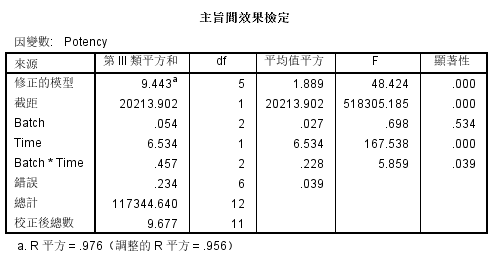R Code

R Result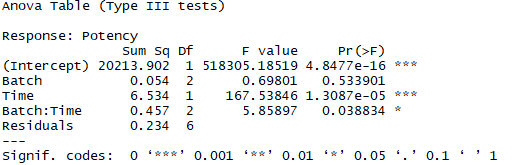#### 系数表

SPSS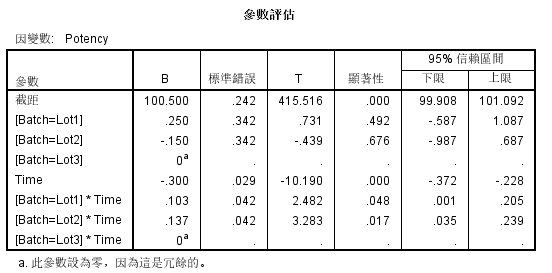R Code

td_fit2<-lm(Potency~Batch*Time,data=td,contrasts=list(Batch=“contr.helmert”)) library(car) print(Anova(td_fit2,type=“III”),digits=7) #drop1(td_fit2,~.,test=“F”)

R Result